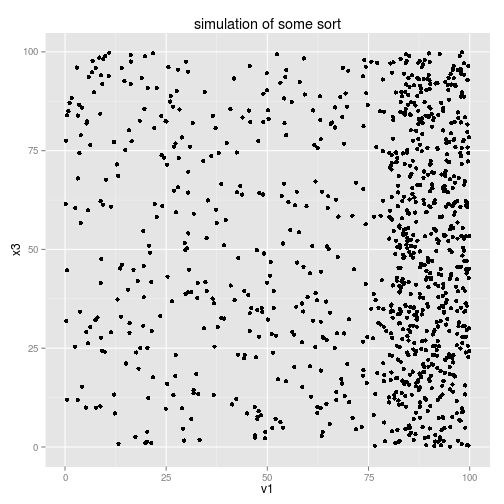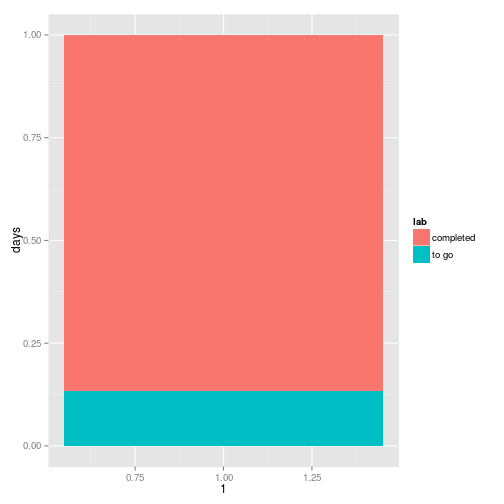# Digital Data Collection - programming extras

Rolf Fredheim and Yulia Shenderovich
University of Cambridge

24/02/2015

### Variables

uni  <-  "The University of Cambridge"
uni

 "The University of Cambridge"


### Paying tax:

#9400 tax free
(20000-9440)*20/100

 2112

#OR:
wage <- 20000
taxFree <- 9400
rate <- 20
(wage-taxFree)*rate/100

 2120


plusOne <- function(x){
return(x+1)
}

plusOne2 <- function(num){
return(num+1)
}


• Curly brackets {} include the code to be executed
• Normal brackets () contain a list of variables
    plusOne(8)

 9

    plusOne2(10)

 11

  plusOne2(num=5)

 6

  #plusOne2(wrongVar=2)


### Simple loops

for (number in 1:5){
print (number)
}

 1
 2
 3
 4
 5


### Looping over functions

a <- c(1,2,3,4,5)
for (value in a){
print (
plusOne(value)
)
}

 2
 3
 4
 5
 6

listOfNumbers <- c(1,2,3,4,5)
for (number in listOfNumbers){
print (
number+1
)
}

 2
 3
 4
 5
 6


### More loops

a <- c(1,2,3,4,5)
a #The first number in the vector

 1

a #The fourth number in the vector

 4

for (i in 1:length(a)){
print (
plusOne(a[i])
)
}

 2
 3
 4
 5
 6


### Functions without variables

printName <- function(){
print ("My name is Rolf Fredheim")
}

printName()

 "My name is Rolf Fredheim"


This is a useless function. But sometimes, if we have many lines of code requiring no particular input, it can be useful to file them away like this.

### e.g. for simulations

sillySimulation <- function(){
x1 <- runif(500,80,100)
x2 <- runif(500,0,100)
v1 <- c(x1,x2)

x3 <- runif(1000,0,100)

df <- data.frame(v1,x3)
require(ggplot2)

print(ggplot(df, aes(v1,x3))+geom_point()+ggtitle("simulation of some sort"))
}


Just as this slide hides the code on the previous slide, so the function hides the underlying code.

sillySimulation()### Inserting variables

Let's hammer home how to use variables

what variables could we add to the function below?

desperateTimes <- function(){
print(paste0("Rolf is struggling to finish his PhD on time. Time remaining: 6 months"))
}


### Name

desperateTimes <- function(name){
print(paste0(name ," is struggling to finish his PhD on time. Time remaining: 6 months"))
}
desperateTimes(name="Tom")

 "Tom is struggling to finish his PhD on time. Time remaining: 6 months"


### Gender

we specify a default value

desperateTimes <- function(name,gender="m"){
if(gender=="m"){
pronoun="his"
}else{
pronoun="her"
}

print(paste0(name ," is struggling to finish ",pronoun," PhD on time. Time remaining: 6 months"))
}
desperateTimes(name="Tanya",gender="f")

 "Tanya is struggling to finish her PhD on time. Time remaining: 6 months"


Is this a good function? Why (not)?

### degree

desperateTimes <- function(name,gender="m",degree){
if(gender=="m"){
pronoun="his"
}else{
pronoun="her"
}

print(paste0(name ," is struggling to finish ",pronoun," ",degree," on time. Time remaining: 6 months"))
}
desperateTimes(name="Rolf",gender="m","Mphil")

 "Rolf is struggling to finish his Mphil on time. Time remaining: 6 months"


require(lubridate)
require(ggplot2)
print(daysLeft)

Time difference of 190 days

print(paste0("Rolf is struggling to finish his PhD on time. Days remaining: ", as.numeric(daysLeft)))

 "Rolf is struggling to finish his PhD on time. Days remaining: 190"


### part2

print(paste0("Percentage to go: ",round(as.numeric(daysLeft)/as.numeric(totDays)*100)))

 "Percentage to go: 13"

df <- data.frame(days=c(daysLeft,totDays-daysLeft),lab=c("to go","completed"))
ggplot(df,aes(1,days,fill=lab))+geom_bar(stat="identity",position="fill")We could put all this code in a function, and forget about it

timeToWorry <- function(){
require(lubridate)
print(daysLeft)
print(paste0("Rolf is struggling to finish his PhD on time. Days remaining: ", as.numeric(daysLeft)))
print(paste0("Percentage to go: ",round(as.numeric(daysLeft)/as.numeric(totDays)*100)))
df <- data.frame(days=c(daysLeft,totDays-daysLeft),lab=c("to go","completed"))
ggplot(df,aes(1,days,fill=lab))+geom_bar(stat="identity",position="fill")
}


### File it away until in need of a reminder

timeToWorry()

Time difference of 190 days
 "Rolf is struggling to finish his PhD on time. Days remaining: 190"
 "Percentage to go: 13"